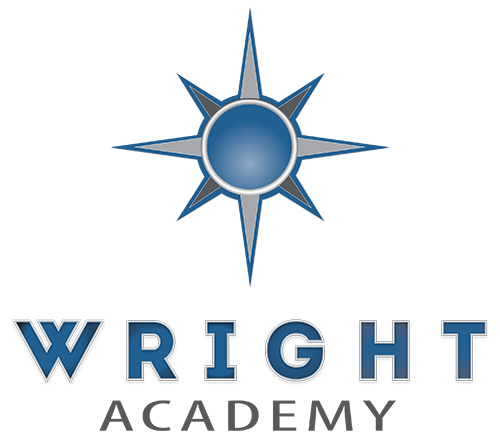## Mathematics

Algebra

Algebra topics include recognizing and developing patterns using tables, graphs, and equations. In addition, students will explore operations on algebraic expressions and apply mathematical properties to algebraic equations. The main objective of Algebra is to learn to analyze and solve various problems.

Geometry

Geometry deals with the measurements, properties, and relationships of points, lines, angles, surfaces, and solids. The goal of this course is to improve reasoning skills and spatial awareness.

Consumer Math

Consumer math focuses on understanding math in everyday life. Students will be learning the skills and understanding of using math in the post-secondary world. This is to prepare students to be independent and financially responsible.